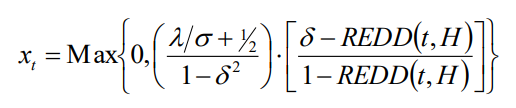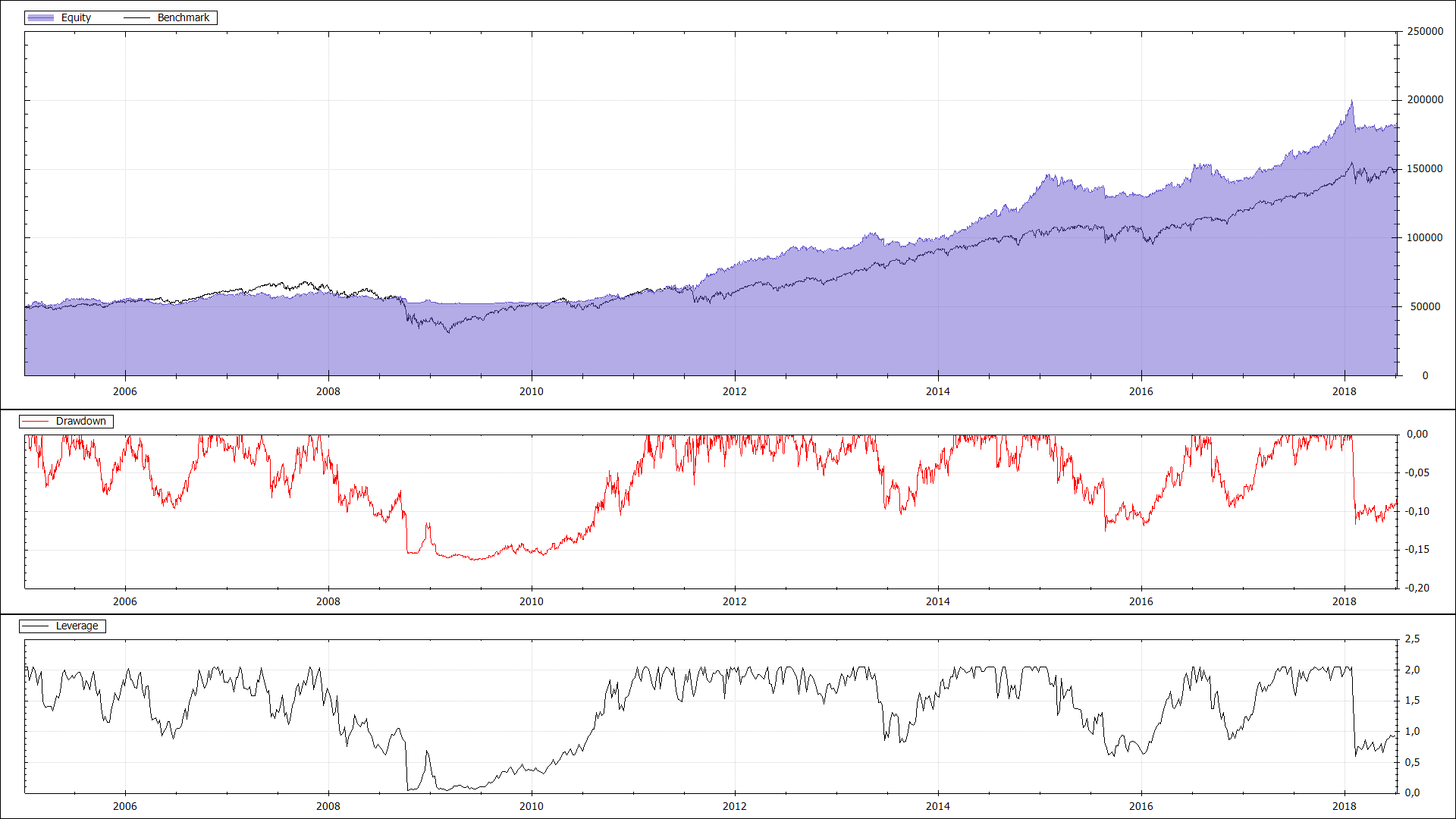# Controlling Drawdown

I read the paper “Optimal Portfolio Strategy to Control Maximum Drawdown” and implemented the ideas presented in it in my trading strategy to controll the maximum drawdown.

The paper Optimal Portfolio Strategy to Control Maximum Drawdown describes a formula one can use to limit the drawdown of a trading strategy to a desired maximum percentage. The formula not only allows to controll the drawdown but also controlls the maximum leverage factor to use while still maintaining the maximum drawdown.The meanings of the individual variables are described in the paper in detail. The result xt will tell us the leverage factor to use.

I implemented this in my C# code in the following way:

``````// Reference: Optimal Portfolio Strategy to Control Maximum Drawdown
// https://papers.ssrn.com/sol3/papers.cfm?abstract_id=2053854
public class DrawdownController
{
/// <summary>
/// Gets or sets the expected future kelly fraction (= Sharpe / Volatility)
/// </summary>
public double KellyFraction { get; set; } = 1.0;

/// <summary>
/// Gets or sets the maximum allowed drawdown. Range [0, 1].
/// </summary>
public double MaxDrawdown { get; set; } = 0.20;

/// <summary>
/// Calculates the leverage factor to use to make sure drawdown stays under MaxAllowedDrawdown.
/// </summary>
/// <param name="currentDrawdown">The current portfolio drawdown.</param>
/// <returns>The leverage factor to use.</returns>
public double Calculate(double currentDrawdown)
{
var timing = (MaxDrawdown - currentDrawdown) / (1.0 - currentDrawdown);
var leverage = (KellyFraction /* + 0.5 */) / (1.0 - MaxDrawdown * MaxDrawdown);
return Math.Max(0.0, leverage * timing);
}
}
``````

I left out the +0.5 in the formula since I did not completely understand why it was there and I wanted KellyFraction to be the maximum value that could ever come out of the formula.

The KellyFraction is a parameter that needs to be estimated and is just the expected future Sharpe Ratio divided by the expected volatility.

The currentDrawdown parameter is the REDD in the formula and has to be computed from the equity curve in every step.

``````maxEquity = Math.Max(maxEquity, Portfolio.Equity);
...
var currentDrawdown = 1.0 - Portfolio.Equity / maxEquity;
var leverage = drawdownController.Calculate(currentDrawdown);
``````

In some cases the drawdown reaches its limit and then it is possible to get stuck there, since then the leverage is very low or even zero. In order to solve this issue i modify the maxEquity variable in my code, so that with time the computed drawdown will be lower and trading starts again.

``````maxEquity = Portfolio.Equity + (maxEquity - Portfolio.Equity) * 0.9975;
``````

The equity curve of a SPY/TLT minimum variance portfolio with a 15% drawdown limit looks like this:During the financial crisis the drawdown limit is reached. After 2010 it recovers and then outperforms the S&P500 since it uses leverage.